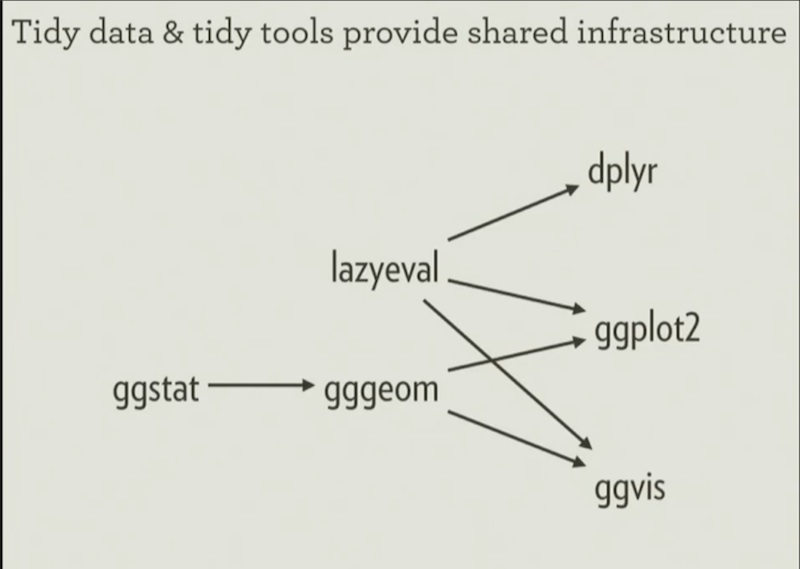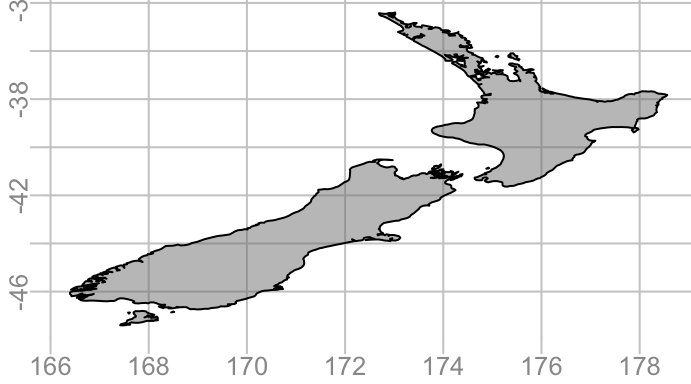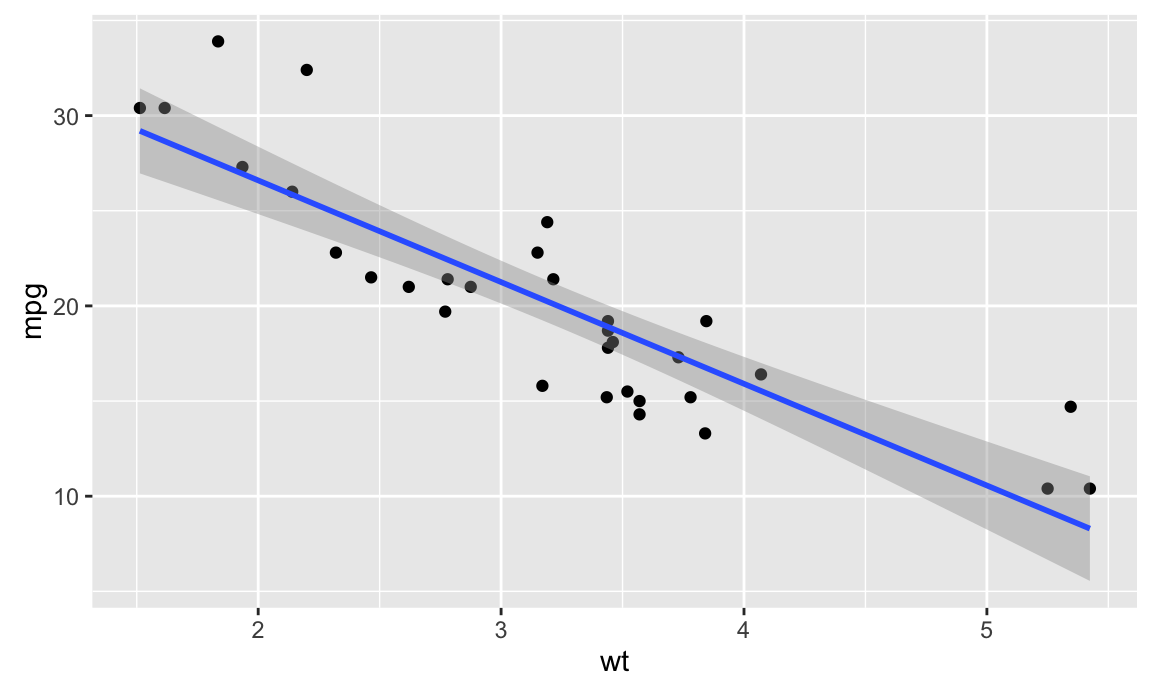July 14, 2016

## UseR 2016

• Happened at the end of June
• Most talks are available online

## Tenets of the tidyverse

1. Uniform data structures
2. Uniform APIs## Uniform data structures

• Data goes in a data frame
• Variables go in columns

Tibbles are lazy, surly data frames

library(tibble)

tibble::data_frame(x = 1:3, y = list(1:5, 1:10, 1:20))
## # A tibble: 3 x 2
##       x          y
##   <int>     <list>
## 1     1  <int >
## 2     2 <int >
## 3     3 <int >

## Uniform APIs

• A set of tidy tools for manipulating tidy data## gggeom

head(nz)
##           x_         y_         island
## 1 <dbl> <dbl>          North
## 2 <dbl> <dbl>          South
## 3  <dbl>  <dbl>        Stewart
## 4  <dbl>  <dbl>  Great.Barrier
## 5  <dbl>  <dbl>     Resolution
## 6   <dbl>   <dbl> Little.Barrier
## Geometry: geom_polygon
plot(nz)## ggstat

Extracts stats needed by viz tools and makes them fast

ggplot(mtcars, aes(x = wt, y = mpg)) +
geom_point() +
geom_smooth(method = "lm")## lazyeval

R is not just a programming language, it's an interactive data environment.

# Interactive use
mtcars %>% filter(cyl == 8)

# Doesn't work
x <- cyl == 8
mtcars %>% filter(x)

# Also doesn't work
var <- cyl
mtcars %>% filter(var == 8)

NSE violates referential transperancy

## lazyeval

Formula notation is used as a quoting operator

mtcars %>% filter_(~ cyl == 8)

# Works now
x <- ~ cyl == 8
mtcars %>% filter_(x)

# Will work soon
var <- ~ cyl
mtcars %>% filter_(~ uq(var) == 8)

Look for lazyeval throughout the future tidyverse